# node爬取数据实例：聊聊怎么抓取小说章节

node怎么爬取数据？下面本篇文章给大家分享一个node爬虫实例，聊聊利用node抓取小说章节的方法，希望对大家有所帮助！`node`中对于网站的请求，本身就存在`http``https`库，内部含有`request`请求方法。

```request = https.request(TestUrl, { encoding:'utf-8' }, (res)=>{
let chunks = ''
res.on('data', (chunk)=>{
chunks += chunk
})
res.on('end',function(){
console.log('请求结束');
})
})```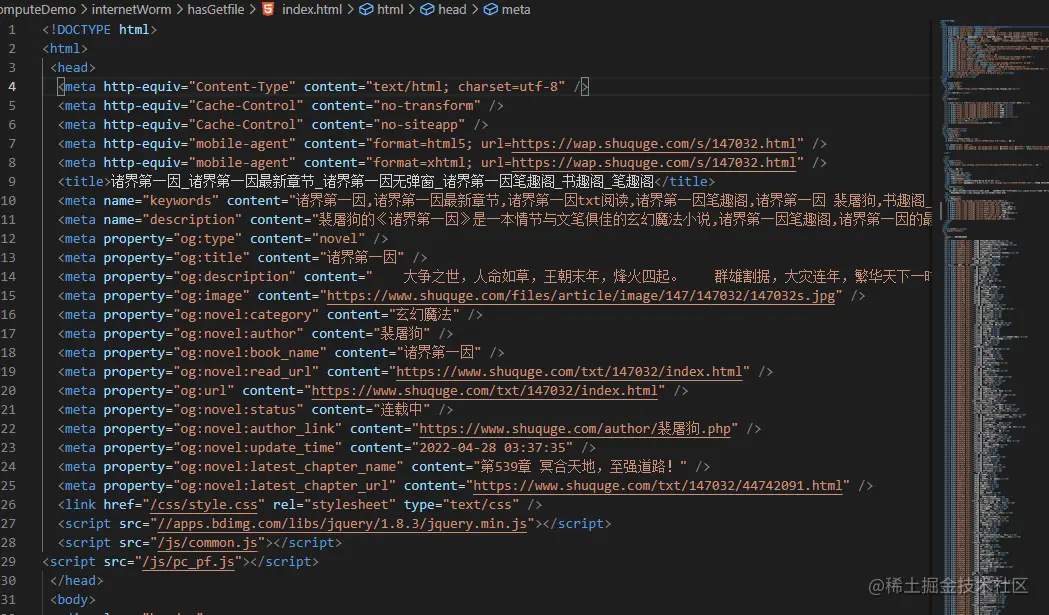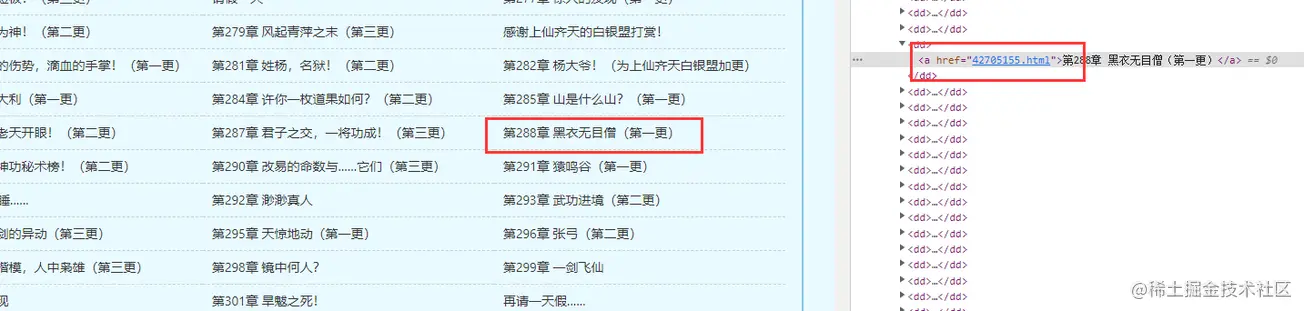## cheerio库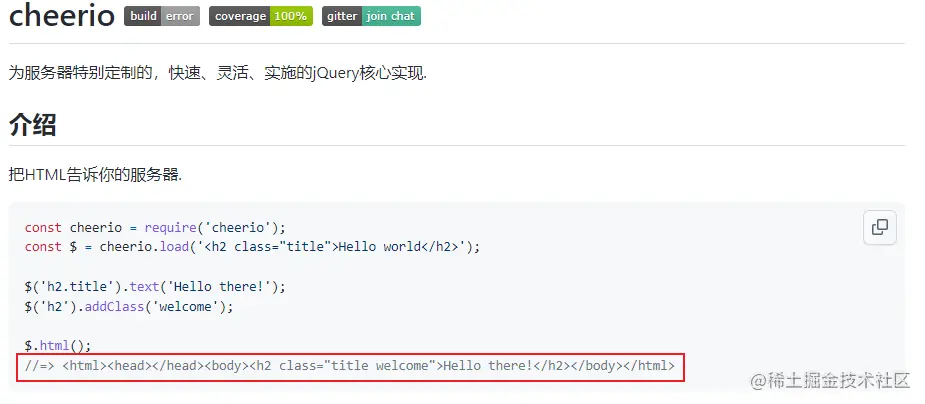## 使用cheerio解析HTML

cheerio解析html时，获取dom节点的方式与`jquery`相似。

```const fs = require('fs')
const cheerio = require('cheerio');

// 引入读取方法
const { getFile, writeFun } = require('./requestNovel')

let hasIndexPromise = getFile('./hasGetfile/index.html');

let bookArray = [];

hasIndexPromise.then((res)=>{
let htmlstr = res;

\$(".listmain dl dd a").map((index, item)=>{
let name = \$(item).text(), href = 'https://www.shuquge.com/txt/147032/' + \$(item).attr('href')
if (index > 11){
bookArray.push({ name, href })
}

})
// console.log(bookArray)
writeFun('./hasGetfile/hrefList.txt', JSON.stringify(bookArray), 'w')
})```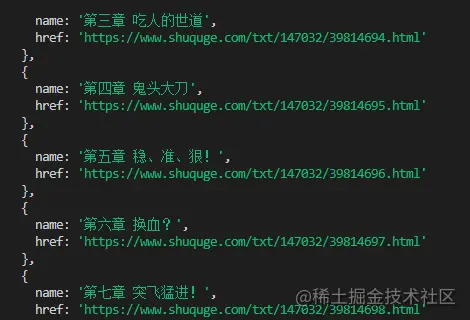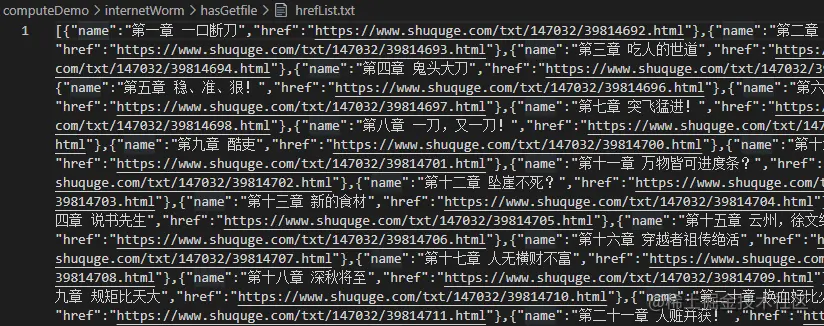```// 爬取某一章节的内容方法
function getOneChapter(n) {
return new Promise((resolve, reject)=>{
if (n >= bookArray.length) {
reject('未能找到')
}
let name = bookArray[n].name;
request = https.request(bookArray[n].href, { encoding:'gbk' }, (res)=>{
let html = ''
res.on('data', chunk=>{
html += chunk;
})
res.on('end', ()=>{
let content = \$("#content").text();
if (content) {
// 写成txt
writeFun(`./hasGetfile/\${name}.txt`, content, 'w')
resolve(content);
} else {
reject('未能找到')
}
})
})
request.end();
})
}

getOneChapter(10)```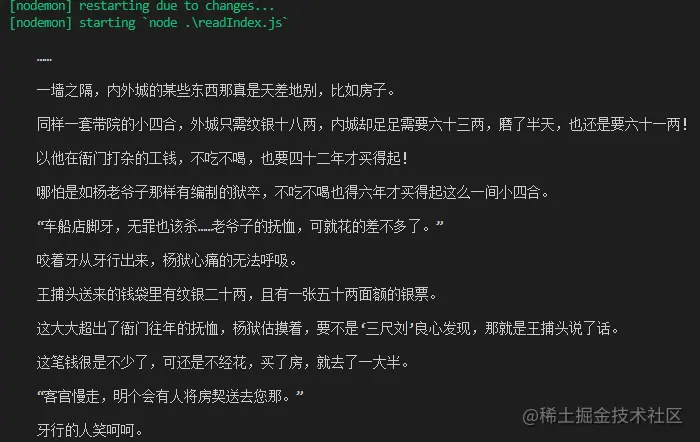```const express = require('express');
const IO = express();
const { getAllChapter, getOneChapter } = require('./readIndex')
// 获取章节超链接链表
getAllChapter();

IO.use('/book',function(req, res) {
// 参数
let query = req.query;
if (query.n) {
// 获取某一章节数据
let promise = getOneChapter(parseInt(query.n - 1));
promise.then((d)=>{
res.json({ d: d })
}, (d)=>{
res.json({ d: d })
})
} else {
res.json({ d: 404 })
}

})

//服务器本地主机的数字
IO.listen('7001',function(){
console.log("启动了。。。");
})```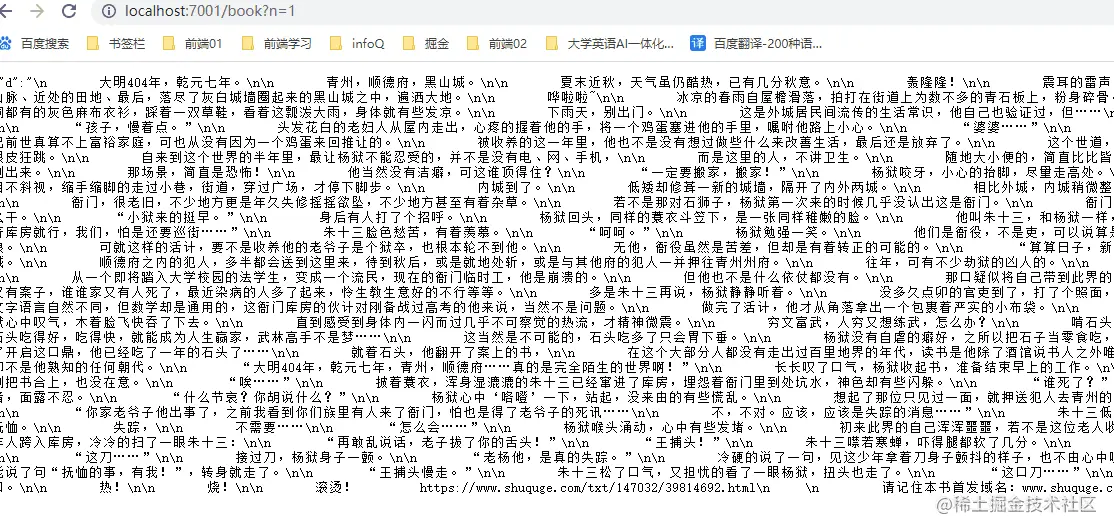1/1